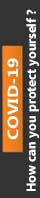We're Open

0% Plagiarism Guaranteed & Custom Written

# 1a) Use the sample standard deviation and sample correlation coefficients to calculate the 5-day VaR (5th percentile) using the VCV method and compare this with the Single Index Model approach (SIM). Briefly comment

Question 1: VaR, VCV & SIM You can use US stocks or UK stocks in the following. Obtain (recent, eg. 1000 or more days) of daily stock price data on 3 US stocks (A-C) from different sectors (e.g. retail or financial, or energy, etc). Assume you hold \$100,000, \$90,000 and \$80,000 in each stock, respectively.

1a) Use the sample standard deviation and sample correlation coefficients to calculate the 5-day VaR (5th percentile) using the VCV method and compare this with the Single Index Model approach (SIM). Briefly comment.

1b) i) Use the SIM model and the EWMA approach (with the “weighting ” factor,  =0.97) to assess the accuracy of the 1-day VaR forecast (at the 5th percentile), over a chosen data sample. ii) Briefly state changes required to assess the above VaR forecast over 5 days.

Question 2: VaR: Monte Carlo Simulation (MCS) and Historical Simulation ( HS) Get recent (5 years?) daily stock price data on ONE major company (eg. Apple, Microsoft , BA Easyjet, JPMorgan, Citigroup). Assume you hold ATM (European) call options on “stock-A”, with maturity 1 year. (Each call delivers one stock). The risk-free rate is 3%pa.(continuously compounded). Assume you invest \$10,000 in the call.

a) Use Black-Scholes to calculate the price of the call on stock-A.

b) Use MCS to calculate the 30-day VaR (5th percentile) for your position in the call options (on stock-A). Calculate the VaR using:

i) Black-Scholes

ii) the “delta approximation” for the change in the call premium Briefly comment on the method and potential accuracy of the 2 approaches.

Historical Simulation

c) Use Black-Scholes for the price of the call on “stock-A”. Then use the historical simulation method to obtain the 1-day VaR(5th percentile) for \$10,000 in the calls. Briefly comment on the results in (c), relative to those in b)

QUESTION 5. FX Derivatives The current USD-GBP spot FX rate is S=1.15 USD per GBP. Interest rates in the US (“domestic”) and UK (“foreign”) are rUS = 2%pa and rUK =1%pa (continuously compounded). The volatility of the spot FX rate is σ = 20% pa. Futures and (European) ATM option contracts are available with a maturity of T = 6 months. Each futures and options contract is for delivery of GBP125,000.

a) Calculate the futures price, the price and delta of the call and put options using Black-Scholes. Check the option prices by using put-call parity

b) The US firm “BigTrump” is importing 10 Rolls-Royce cars (each of which cost £125,000) with delivery and payment in GBP in 6 months time. BigTrump is worried about an increase in the USD cost of its imports. What are the outcomes in 6 months time, if today BigTrump i) does nothing ii) uses appropriate futures (and closes out one day before maturity) iii) uses the appropriate option contract Assume the outcome at T for the spot-FX rate is either ST = 1.2 USD per GBP or ST = 1.05 USD per GBP. Briefly comment.

100% Plagiarism Free & Custom Written,International House, 12 Constance Street, London, United Kingdom,
E16 2DQ

## STILL NOT CONVINCED?

We've produced some samples of what you can expect from our Academic Writing Service - these are created by our writers to show you the kind of high-quality work you'll receive. Take a look for yourself!FLAT 25% OFF ON EVERY ORDER.Use "FLAT25" as your promo code during checkout